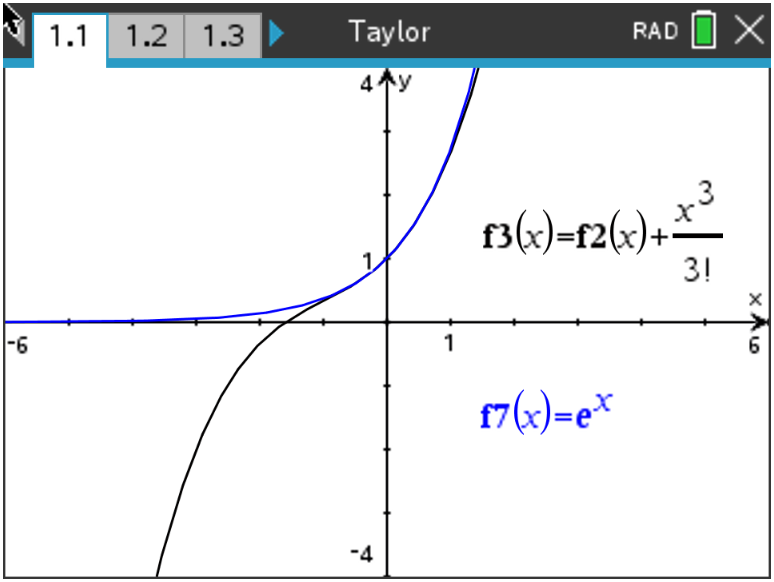# Activities

•• ##### Subject Area

• Math: Calculus: Series and Taylor Polynomials

9-12

50 Minutes

• ##### Device
•TI-Nspire™ CX/CX II
•TI-Nspire™ CX CAS/CX II CAS
• ##### Software

TI-Nspire™ CX
TI-Nspire™ CX CAS

5.0

## Taylor Polynomials Exploration

Updated on 05/11/2021#### Activity Overview

In this activity, students will examine another kind of polynomial approximation that is a generalization of the tangent line approximation. These polynomials are called Taylor polynomials.

#### Objectives

Students will:

• Define a Taylor polynomial approximation to a function f of degree n about a point x = a.
• Graph convergence of Taylor polynomials.
• Use Taylor polynomials to approximate function values.

#### Vocabulary

• Taylor polynomial
• factorial
• derivatives

#### About the Lesson

Taylor polynomial approximations are introduced as generalizations of tangent line approximations. The graphing handheld is used as a tool to graph Taylor polynomial approximations of functions. Taylor polynomials are also used to approximate specific function values. In this activity, students will examine another kind of polynomial approximation that is a generalization of the tangent line approximation. These polynomials are called Taylor polynomials.